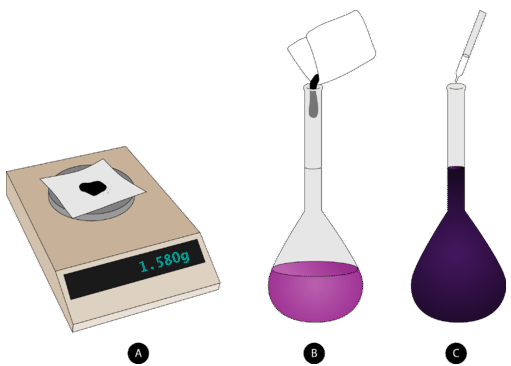# 16.9: Preparing Solutions

Back in the "good old days" (whenever that really was), many cooks didn't bother with careful measurements. They "just knew" how much flour to use or how much water to add. Most of us need somewhat more precise ways to measure when we cook. Chemists are very careful when they prepare solutions since the results of their experiments need to be quantitative. "Just knowing" is not accurate enough for scientific purposes.

## Preparing Solutions

If you are attempting to prepare $$1.00 \: \text{L}$$ of a $$1.00 \: \text{M}$$ solution of $$\ce{NaCl}$$, you would obtain $$58.44 \: \text{g}$$ of sodium chloride. However you cannot simply add the sodium chloride to $$1.00 \: \text{L}$$ of water. After the solute dissolves, the volume of the solution will be slightly greater than a liter because the hydrated sodium and chloride ions take up space in the solution. Instead, a volumetric flask needs to be used. Volumetric flasks come in a variety of sizes (see image below) and are designed to allow a chemist to prepare a solution only of one specific volume.

In other words, you cannot use a 1-liter volumetric flask to make $$500 \: \text{mL}$$ of a solution. It can only be used to prepare 1 liter of a solution. The steps to follow when preparing a solution with a 1-liter volumetric flask are outlined below and shown in the figure below.

1. The appropriate mass of solute is weighed out and added to volumetric flask that has been about half-filled with distilled water.

2. The solution is swirled until all of the solute dissolves.

3. More distilled water is carefully added up to the line etched on the neck of the flask.

4. The flask is capped and inverted several times to completely mix.Figure 16.9.2: Steps to follow in preparing a solution of known molarity: (A) weigh out correct mass of solute, (B) dissolve into solvent in a volumetric flask, and (C) add solvent to the fill line on the flask and mix.

## Summary

• Steps for the preparation of a solution are outlined.

## Contributors

• CK-12 Foundation by Sharon Bewick, Richard Parsons, Therese Forsythe, Shonna Robinson, and Jean Dupon.